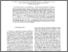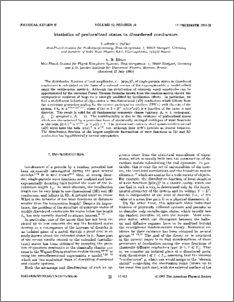Statistics of prelocalized states in disordered conductors

Falko, Vladimir and Efetov, K. B. (1995) Statistics of prelocalized states in disordered conductors. Physical review B, 52 (24). pp. 17413-17429. ISSN 0163-1829Preview
PDF (PhysRevB.52.17413)
PhysRevB.52.17413.pdf - Published Version

Abstract

The distribution function of local amplitudes, t = \psi(r(0))\(2), of single-particle states in disordered conductors is calculated on the basis of a reduced version of the supersymmetric sigma model solved using the saddle-point method. Although the distribution of relatively small amplitudes can be approximated by the universal Porter-Thomas formulas known from the random-matrix theory, the asymptotical statistics of large t's is strongly modified by localization effects. In particular, we find a multifractal behavior of eigenstates in two-dimensional (2D) conductors which follows from the noninteger power-law scaling for the inverse participation numbers (IPN's) with the size of the system, Vt(n) proportional to L(-(n-1)d*(n)), where d*(n) = 2 - beta(-1)n/(4 pi nu D) is a function of the index n and disorder. The result is valid for all fundamental symmetry classes (unitary, beta(u) = 1; orthogonal, beta(o) = 1/2; symplectic, beta(s) = 2). The multifractality is due to the existence of prelocalized states which are characterized by a power-law form of statistically averaged envelopes of wave functions at the tails, \psi(t)(r)\(2) proportional to r(-2 mu), mu = mu(t) < 1. The prelocalized states in short quasi-one-dimensional (1D) wires have the tails \psi(x)\(2) proportional to x(-2), too, although their IPN's indicate no fractal behavior. The distribution function of the largest-amplitude fluctuations of wave functions in 2D and 3D conductors has logarithmically normal asymptotics.

Item Type:
Journal Article
Journal or Publication Title:
Physical review B
© 1995 The American Physical Society
Uncontrolled Keywords:
/dk/atira/pure/researchoutput/libraryofcongress/qc
Subjects:
Departments:
ID Code:
57077
Deposited By:
Deposited On:
05 Sep 2012 16:39
Refereed?:
Yes
Published?:
Published